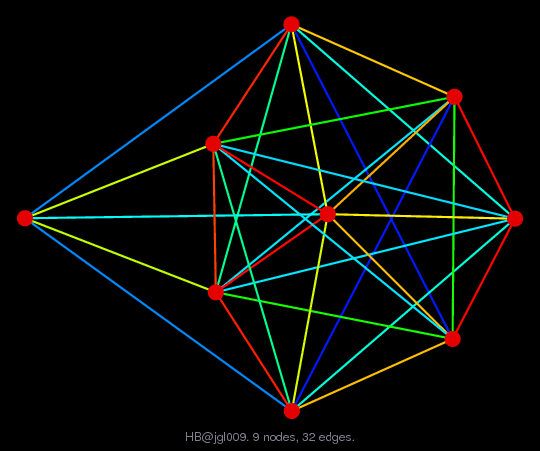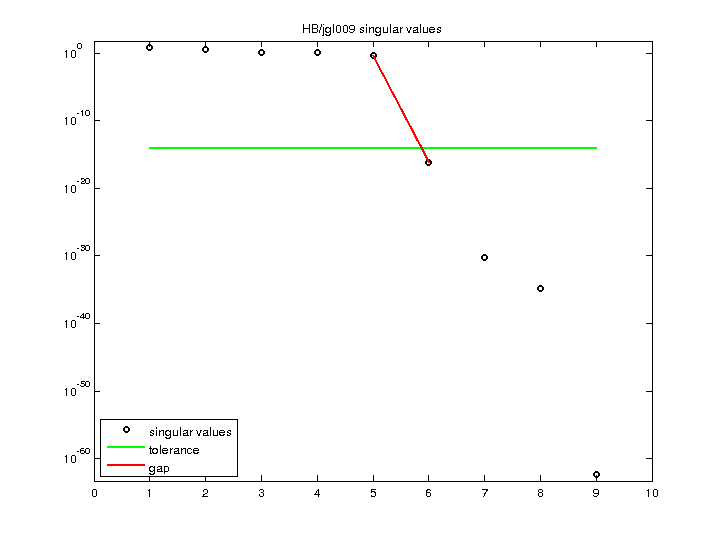Matrix: HB/jgl009

Description: U JOHN G. LEWIS P4 COUNTEREXAMPLE WHICH REQUIRES FILL IN SPIKES(bipartite graph drawing) (graph drawing of A+A')• Matrix group: HB
• download as a MATLAB mat-file, file size: 521 bytes. Use UFget(185) or UFget('HB/jgl009') in MATLAB.

 Matrix properties number of rows 9 number of columns 9 nonzeros 50 structural full rank? yes structural rank 9 # of blocks from dmperm 1 # strongly connected comp. 1 explicit zero entries 0 nonzero pattern symmetry 48% numeric value symmetry 48% type binary structure unsymmetric Cholesky candidate? no positive definite? no

 author J. Lewis editor I. Duff, R. Grimes, J. Lewis date 1983 kind counter-example problem 2D/3D problem? no

 Ordering statistics: result nnz(chol(P*(A+A'+s*I)*P')) with AMD 42 Cholesky flop count 2.4e+02 nnz(L+U), no partial pivoting, with AMD 75 nnz(V) for QR, upper bound nnz(L) for LU, with COLAMD 44 nnz(R) for QR, upper bound nnz(U) for LU, with COLAMD 45

 SVD-based statistics: norm(A) 6.10129 min(svd(A)) 4.86173e-63 cond(A) 1.25496e+63 rank(A) 5 sprank(A)-rank(A) 4 null space dimension 4 full numerical rank? no singular value gap 6.02749e+15

 singular values (MAT file): click here SVD method used: s = svd (full (A)) status: ok